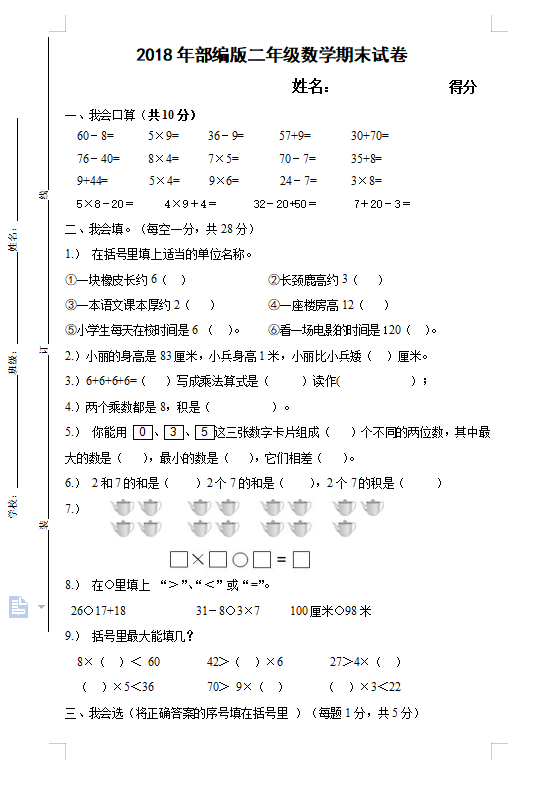2018年部编版小学二年级数学期末试卷

姓名：              得分

60－8=      5×9=     36－9=      57+9=       30+70=

76－40=     8×4=     7×5=       70－7=      35+8=

9+44=       5×4=     9×6=       24－7=      3×8=

5×8－20＝     4×9＋4＝      32－20+50＝      7＋20－3＝